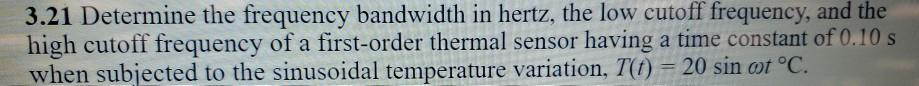# Question Solved1 AnswerPLEASE EXPLAIN EVERY STEP CLEARLY. THANK YOU 3.21 Determine the frequency bandwidth in hertz, the low cutoff frequency, and the high cutoff frequency of a first-order thermal sensor having a time constant of 0.10 s when subjected to the sinusoidal temperature variation, T(t) = 20 sin otºC.PHRSFP The Asker · Mechanical EngineeringTHANK YOU

Transcribed Image Text: 3.21 Determine the frequency bandwidth in hertz, the low cutoff frequency, and the high cutoff frequency of a first-order thermal sensor having a time constant of 0.10 s when subjected to the sinusoidal temperature variation, T(t) = 20 sin otºC.
More
Transcribed Image Text: 3.21 Determine the frequency bandwidth in hertz, the low cutoff frequency, and the high cutoff frequency of a first-order thermal sensor having a time constant of 0.10 s when subjected to the sinusoidal temperature variation, T(t) = 20 sin otºC.